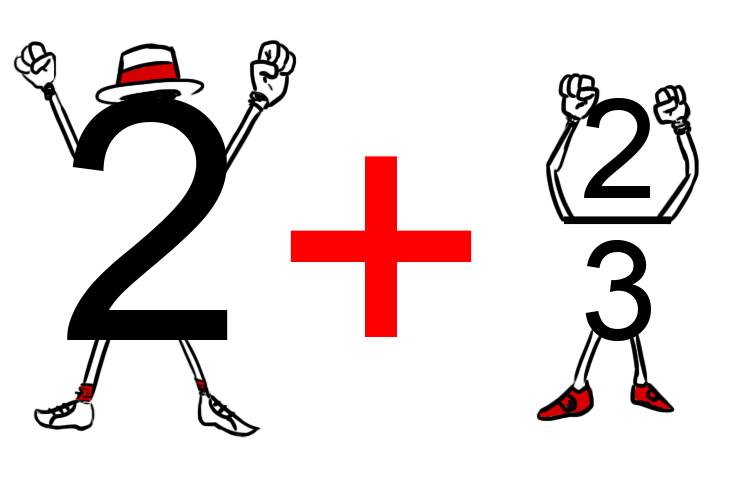# Convert between a mixed number and an improper fraction

We know a mixed number is a whole number (integer) and a fraction.

Improper fractions are fractions with a bigger number on the top than the bottom.

Example

Mixed number is 2\2/3

Improper fraction is 8/3

But to convert between 2\2/3 to 8/3 remember that 2\2/3 really means:So 2\2/3 is really 2+2/3

4\3/8 is really 4+3/8

12\3/13 is really 12+3/13

To convert between a mixed number and an improper number do exactly what it says

Example 1

Convert 2\2/3 to an improper fraction

2\2/3=2+2/3

And in adding think 1/2

we know 1/2+1/2=1

which must be (1+1)/2=1

If adding is the aim the bottom number must be the same

And,

Multiply the top and bottom of each fraction by the other fraction's bottom number.

Therefore 2+2/3=2/1+2/3=2/1times3/3+2/3times1/1

2/1times3/3+2/3times1/1=6/3+2/3

6/3+2/3=(6+2)/3=8/3

Answer: 2\2/3=8/3

Example 2

Convert 4\1/3 to an improper fraction.

We know 4\1/3=4+1/3

And in adding think 1/2 i.e. 1/2+1/2=1

which must be (1+1)/2 (the bottom number must be the same)

If adding is the aim the bottom number must be the same

And,

Multiply the top and bottom of each fraction by the other fraction's bottom number.

therefore 4+1/3=4/1+1/3=4/1times3/3+1/3times1/1

4/1times3/3+1/3times1/1=12/3+1/3

12/3+1/3=(12+1)/3=13/3

Answer: 4\1/3=13/3

Example 3

Convert 2\5/6 to an improper fraction.

We know 2\5/6=2+5/6

And in adding think 1/2 i.e. 1/2+1/2=1

which must be (1+1)/2 (the bottom number must be the same)

If adding is the aim the bottom number must be the same

And,

Multiply the top and bottom of each fraction by the other fraction's bottom number.

therefore 2+5/6=2/1+5/6=2/1times6/6+5/6times1/1

2/1times6/6+5/6times1/1=12/6+5/6

12/6+5/6=(12+5)/6=17/6

Answer: 2\5/6=17/6